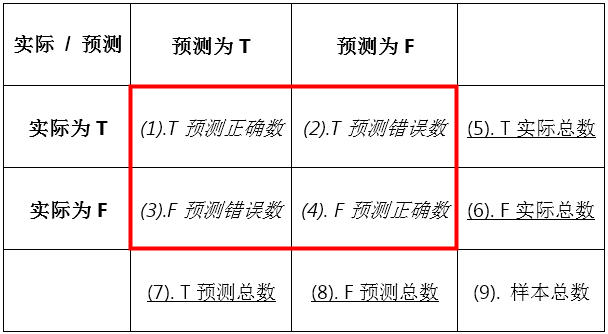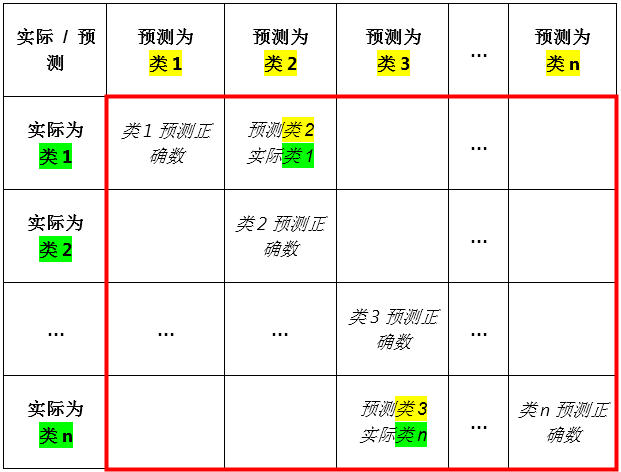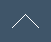### 目前平台处于试运行阶段，如有任何问题或建议，请发送邮件至 service@dataju.cn 或加入QQ群 565136792 联系管理员。

4257次浏览 dataju 于 2016-07-10 发布1、真正，正类预测正确的数量 $$true~positive~(TP),~hit = (1)$$

2、真负，负类预测正确的数量 $$true~negative~(TN),~correct~rejection = (4)$$

3、假正，正类预测错误的数量，统计学上的第一类错误 $$false~positive~(FP),~false~alarm,~Type~I~error = (3)$$

4、假负，负类预测错误的数量，统计学上的第二类错误 $$false~negative~(FN),~miss,~Type~II~error = (2)$$

5、假正召回率 $$false~positive~rate~(FPR),~fall-out = \frac{(3)}{(3)+(4)} = \frac{(3)}{(6)}$$

6、假正精准率 $$false~discovery~rate~(FDR) = \frac{(3)}{(1)+(3)} = \frac{(3)}{(7)}$$

7、准确率、正确率 $$accuracy = \frac{(1) + (4)}{(1)+(2)+(3)+(4)} = \frac{(1) + (4)}{(9)}$$

8、正类精准率 $$positive~predictive~value~(PPV), precision = \frac{(1)}{(1)+(3)} = \frac{(1)}{(7)}$$

9、正类召回率、敏感度 $$sensitivity,~true~positive~rate~(TPR), hit~rate, recall = \frac{(1)}{(1)+(2)} = \frac{(1)}{(5)}$$

10、负类召回率 $$specificity~(SPC),~true~negative~rate (TNR) = \frac{(4)}{(3)+(4)} = \frac{(4)}{(6)}$$

11、负类精准率 $$negative~predictive~value~(NPV) = \frac{(4)}{(2)+(4)} = \frac{(4)}{(8)}$$

12、F值、精准率与召回率的调和平均数 $$F1~score,~harmonic~mean~of~precision~and~recall = \frac{ 2 }{ \frac{1}{precision} + \frac{1}{recall} } = \frac{ 2 \times (1) }{ 2 \times (1) + (2) + (3) } = \frac{ 2 \times (1) }{ (5) + (7) }$$# 应用案例名称 应用案例介绍
1 UCI Iris 数据集支持向量机建模预测鸢花种类 UCI Iris 数据集，采用多分类支持向量机 C-SVC 方法建模，通过鸢花外形数据预测鸢花种类。
2 UCI Iris 数据集决策树建模预测鸢花种类 UCI Iris 数据集，采用多分类支持向量机 C4.5 决策树方法建模，通过鸢花外形数据预测鸢花种类。
3 UCI Iris 数据集K近邻方法建模预测鸢花种类 UCI Iris 数据集，采用多分类 KNN 方法建模，通过鸢花外形数据预测鸢花种类。
4 UCI Germny数据集Logistic Regression建模预测贷款违约 UCI Germny数据集Logistic Regression建模预测贷款违约
5 UCI Germny数据集 Native Bayes 建模预测贷款违约 UCI Germny数据集 Native Bayes 建模预测贷款违约
6 UCI Germny数据集 Nonparallel SVM 建模预测贷款违约 UCI Germny数据集 Nonparallel SVM 建模预测贷款违约
7 UCI Beast-cancer 数据集 SVM 建模预测乳腺癌可能性 UCI Beast-cancer 数据集 SVM 建模预测乳腺癌可能性
8 MNIST数据集SVM手写数字识别建模 MNIST数据集SVM手写数字识别建模

### 目前平台处于试运行阶段，如有任何问题或建议，请发送邮件至 service@dataju.cn 或加入QQ群 565136792 联系管理员。